# PERMISSIBLE STRESSES IN AXIAL TENSION

It is related to the guaranteed minimum yield point of steel with an appropriate factor of safety. Most of the codes assume a factor of safety of 1.67.

The direct stress in axial tension or the effective net area should not exceed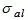given by the equation,

Where,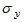= minimum yield stress of steel in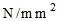(MPa).

Indian Standard IS: 226

It stipulates the following permissible stress in axial tension for steel,

Permissible Combined Stress

When tension members are subjected to both axial loading and bending moment, then the permissible stress is governed by the formula,

Where,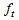= axial tensile stress,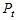= permissible axial stress in tension,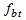= calculated bending stress in tension in the extreme fibre, and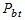= permissible bending stress in tension.